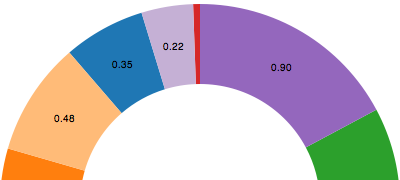Wiki ▸ [[API Reference]] ▸ [[Layouts]] ▸ Pie Layout

The pie layout is a convenience for computing the start and end angles of arcs that comprise a pie or donut chart:You don't need to use the pie layout to create a pie chart; you can just use the arc shape directly if you prefer. The pie layout simply makes it easier to convert an array of data into an array of objects with startAngle and endAngle attributes that range from 0 to 2π, which you can then pass to the arc shape generator.

# d3.layout.pie()

Constructs a new pie function with the default value accessor (number), sort comparator (descending value), start angle (0) and end angle (2π). The returned layout object is both an object and a function. That is: you can call the layout like any other function, and the layout has additional methods that change its behavior. Like other classes in D3, layouts follow the method chaining pattern where setter methods return the layout itself, allowing multiple setters to be invoked in a concise statement.

# pie(values[, index])

Evaluates the pie function on the specified array of values. An optional index may be specified, which is passed along to the start and end angle functions. The return value is an array of arc descriptors:

• value - the data value, returned by the value accessor.
• startAngle - the start angle of the arc in radians.
• endAngle - the end angle of the arc in radians.
• data - the original datum for this arc.

The elements are returned in the original order, matching values, even if a sort order is applied; this preserves the original index of each element in the values array, which is nice if you are using the index to generate a categorical color or other display property.

# pie.value([accessor])

Specifies how to extract a value from the associated data (e.g. sets the accessor function for the pie layout to use); accessor is a function which is invoked on each input value passed to pie, equivalent to calling values.map(accessor) before computing the pie layout. The function is passed two arguments: the current datum and the current index. The default value function is the built-in Number, which is similar to the identity function. If accessor is not specified, returns the current value accessor.

# pie.sort([comparator])

If comparator is specified, sets the sort order of data for the layout using the specified comparator function. Pass null to disable sorting. If comparator is not specified, returns the current sort order. The sort order defaults to descending value. Sorting preserves the index (and z-index) of input values, affecting only the computed angles. The comparator function is invoked for pairs of data elements from the values array passed to pie. Comparator functions may also be implemented using d3.ascending or d3.descending.

# pie.startAngle([angle])

If angle is specified, sets the overall start angle of the pie layout to the specified value in radians. If angle is not specified, returns the current value, which defaults to 0. The start angle can be specified either as a constant or as a function; if a function, it is evaluated once when the pie function is called, being passed the current data and index.

# pie.endAngle([angle])

If angle is specified, sets the overall end angle of the pie layout to the specified value in radians. If angle is not specified, returns the current value, which defaults to 2π. The end angle can be specified either as a constant or as a function; if a function, it is evaluated once when the pie function is called, being passed the current data and index.